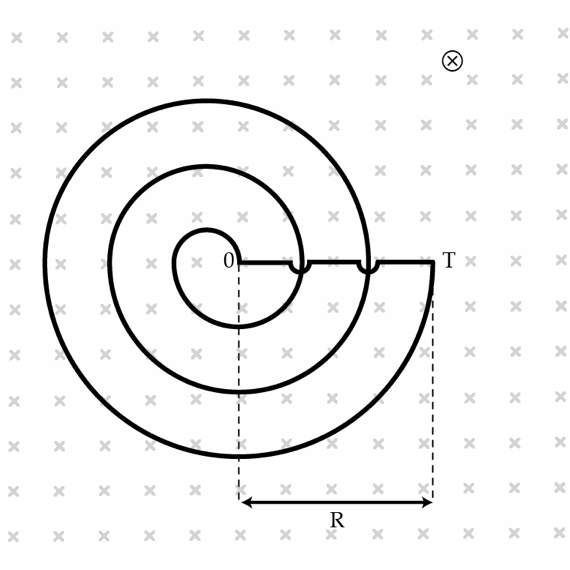# Emf in the Archimedean spiral

A wire is bent into the shape of an planar Archimedean spiral which in polar coordinates is described by the equation $r= b \theta.$ The spiral has $N=100$ turns and outer radius $R=10~\mbox{cm}$ ($R$ is the distance from point O to point T). Note that in the figure below we show a spiral having only 3 turns. The circuit is placed in a homogeneous magnetic field perpendicular to the plane of the spiral. The time dependence of the magnetic field induction is given by $B=B_{0}\cos(\omega t)$ where $B_{0}=1 ~\mu \mbox{T}$ and $\omega=2\times 10^{6}~\mbox{s}^{-1}$. Determine, the amplitude of the emf in Volts induced in the circuit.×

Problem Loading...

Note Loading...

Set Loading...